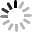NEWS
Home > news > Company news about How to calculate the power of stepping motor?
EVENTS
86-020-36907671
Contact Now

# How to calculate the power of stepping motor?

2023-02-09Theoretically, the power of stepping motor can be calculated when it is running, but it is not scientific in terms of power overall. Because the power consumed by the motor changes when the control speed becomes faster or slower, each time point will generate voltage itself, and the voltage generated at different time points is not exactly the same. The voltage generated by the motor will offset the input voltage at the same time, so the calculated power is only at a certain moment, and cannot represent its whole. So, how to calculate the power of stepping motor, so we use torque to measure it.

Stepping motor is characterized by low torque, and the torque drops sharply after exceeding the rated speed. The relationship between the two is nonlinear. So for a stepper motor, the output power is different at different speeds. Therefore, we mainly refer to the parameter of torque when selecting models. If you must have a thorough understanding of how to calculate the power of stepping motor, you can refer to the following calculation method:

Torque and power are converted as follows: P=Ω · M, because Ω=2 π· n/60, P=2 π nM/60; P is power, unit is watt, Ω is angular velocity per second, unit is radian, n is rotational speed per minute, M is torque unit is newton meters.

Send your inquiry directly to us